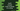# Swift program to convert an array of string to a string## How to convert an array of strings to a string in swift:

It is easy to convert an array of strings to a single string in swift.Swift provides one joined() method that can be used to join all strings in an array. We can also add our own custom separator to separate all values. Below is the method:

``joined(separator:)``

It returns the concatenated value by joining all elements of the array. It inserts the separator between each elements. separator is a sequence.

### Examples:

Let’s consider the below example:

``````let given_arr = ["one", "two", "three", "four"]
print(given_arr.joined())``````

It will print:

``onetwothreefour``

It will create the string without adding any separator.

``````let given_arr = ["one", "two", "three", "four"]
print(given_arr.joined(separator: ","))``````

It will print:

``one,two,three,four``

We can also add one array as the separator if we have array elements:

``````let given_arr = [[1,2], [3,4], [5,6]]
let joined_arr = given_arr.joined(separator: [0,0])

print(Array(joined_arr))``````

It will print:

``[1, 2, 0, 0, 3, 4, 0, 0, 5, 6]``